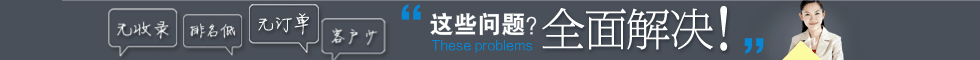91信息网(http://www.97605.com)是一个免费发布信息的B2B平台.通过发布企业信息,免费发布供应信息,企业黄页,产品求购.帮助企业搭建企业信息平台,为中小企业网络营销服务.是企业首选的B2B电子商务网站.

91信息网企业信息发布

I T 数码

• 发信息
• 做推广
• 学经验
• 有疑问

• a
• b
• c
• d
• e
• f
• g
• h
• i
• j
• k
• l
• m
• n
• o
• p
• q
• r
• s
• t
• u
• v
• w
• x
• y
• z